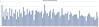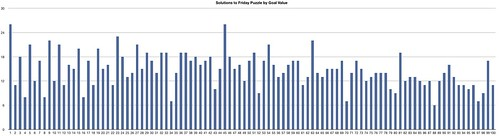American in Spain

# Fun With Arithmetic

July 16, 2010I'm an avid reader of Richard Wiseman's blog, and I find his Friday Puzzles to be particularly fun. Today's puzzle is as follows:

How can you place the arithmetical signs â€˜+\' and â€˜-\' between the consecutive numbers 123456789 so that the end result is 100? So, for example, you could go…..

12+34+56-7-89 , but that would make 6, so that doesn\'t work.

Try to come up with the method that uses the least number of symbols.

My first thought was, "It would be so easy to write a program to try all the possibilities!" So I did. Thirty minutes and 41 lines of javascript code later, I had this: .test-button { text-decoration:none !important; padding: 5px; font-size: 1.2em; background-color: #cfc; color: black !important; border:1px outset #999 !important; } .test-button:hover { background-color: #9f9; border:1px outset #999 !important; } .test-button:active { border-style: inset !important; } .inputs-table { border: 1px solid #ccc; margin-left: 150px; margin-bottom: 20px; } .inputs-table td, .inputs-table th { padding: 5px; } .inputs-table th { text-align:right; } #results { text-align:center; } #results ol li { text-align:left; } #calculationStatus { text-align:center; margin-top:15px; }

How many digits?

2 3 4 5 6 7 8 9

Goal Value

Generate Equations

## Charting Number of Solutions

Once I had the code to quickly generate the solutions for any arbitrary goal value, I decided to see if there was a pattern in the number of solutions between the goal values from 1 to 100. There isn't.Click to see large.

## The Code

It's just a matter of changing the operation in each slot between nothing, + and -, and when you roll back to nothing, you increment the next slot. Javascript's built in eval() function came in very handy to calculate the value of each equation.

function test() { function next(slots, operations) { for (var i = 0; i < slots.length; i++) { if (!slots[i]) { slots[i] = operations; return slots; } else { var index = operations.indexOf(slots[i]); if (index + 1 < operations.length) { slots[i] = operations[index + 1]; return slots; } else delete slots[i]; } } return null; } function makeEquation(slots) { equation = ['1']; for (var i = 0; i < slots.length; i++) { if (slots[i]) equation.push(' ' + slots[i] + ' '); equation.push(i + 2); } return equation.join(''); } var resultsList = document.getElementById('resultsList'); if (resultsList) resultsList.remove(); var operations = ['+', '-']; var slots = new Array(document.getElementById('numDigits').value - 1); var goalValue = document.getElementById('goalValue').value; while (slots != null) { var equation = makeEquation(slots); if (eval(equation) == goalValue) printResult(equation + ' = ' + goalValue); slots = next(slots, operations); } }